Documentation

## Control of a Linear Electric Actuator Using Control System Tuner

This example shows how to use the Control System Tuner app to tune the current and velocity loops in a linear electric actuator with saturation limits.

### Linear Electric Actuator Model

Open the Simulink model of the linear electric actuator:

```open_system('rct_linact') ```The electrical and mechanical components are modeled using Simscape Electrical and Simscape Multibody. The control system consists of two cascaded feedback loops controlling the driving current and angular speed of the DC motor.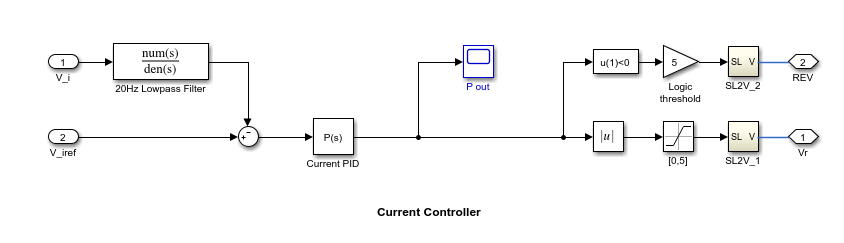Figure 1: Current and Speed Controllers.

Note that the inner-loop (current) controller is a proportional gain while the outer-loop (speed) controller has proportional and integral actions. The output of both controllers is limited to plus/minus 5.

### Design Specifications

We need to tune the proportional and integral gains to respond to a 2000 rpm speed demand in about 0.1 seconds with minimum overshoot. The initial gain settings in the model are P=50 and PI(s)=0.2+0.1/s and the corresponding response is shown in Figure 2. This response is too slow and too sensitive to load disturbances.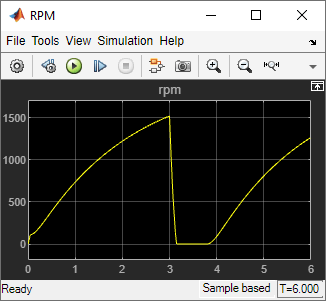Figure 2: Untuned Response.

### Control System Tuning

You can use Control System Tuner to jointly tune both feedback loops. First, open Control System Tuner from the Apps tab.Figure 3: Opening Control System Tuner.

This opens Control System Tuner.Figure 4: Control System Tuner.

You linearize the model at t=0.5 to avoid discontinuities in some derivatives at t=0. You can set the operating point in `Linearize At...`.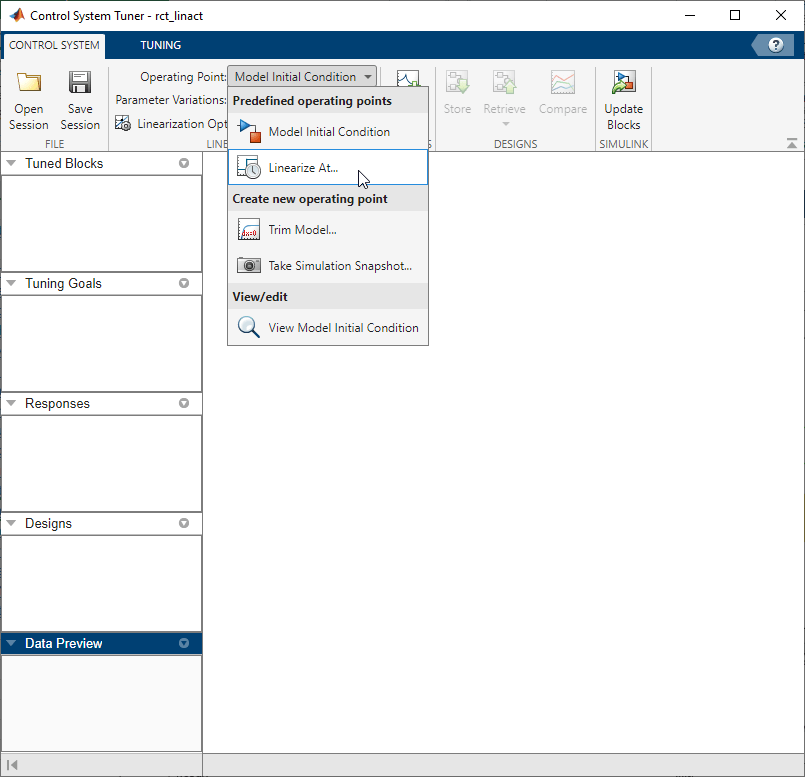Figure 5: Setting Operating Point for Linearization.

Set the linearization snapshot time at t=0.5.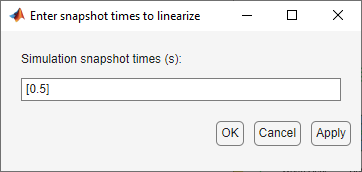Figure 6: Setting the Linearization Snapshot Time.

In order to set the tuned blocks of the control system, open `Select Blocks` from `Tuning` tab.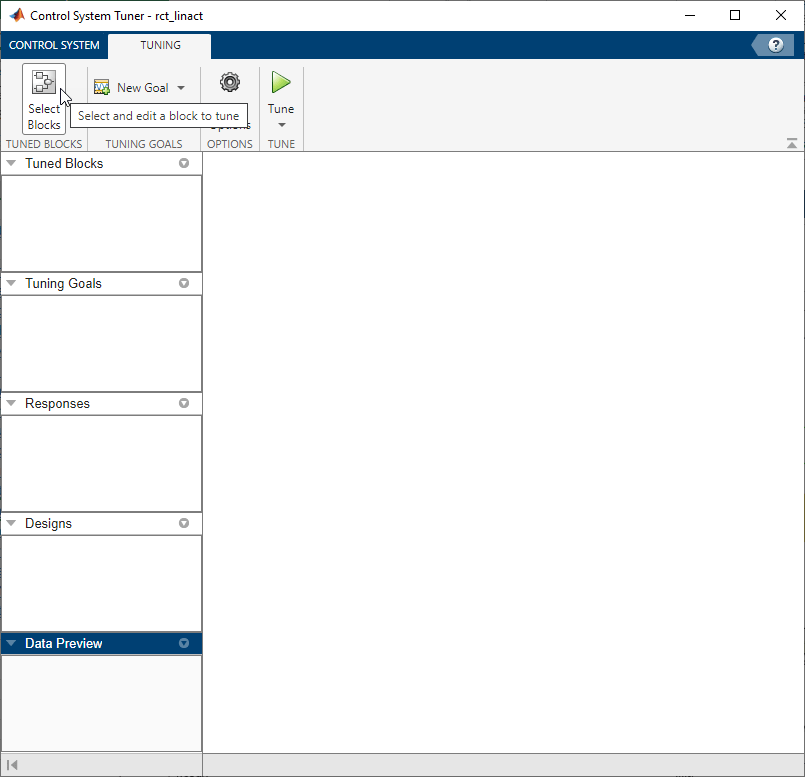Figure 7: Tuning Tab of Control System Tuner.

This shows the editor for tuned blocks where you can `Add Blocks`.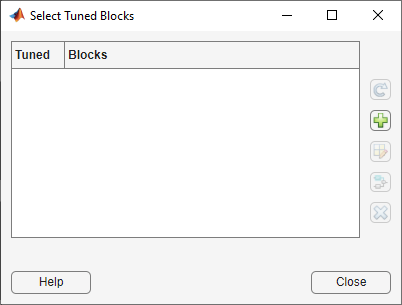Figure 8: Editor for Tuned Blocks.

Set the tuned blocks `Current PID` and `Speed PID` by navigating through the tree on the left.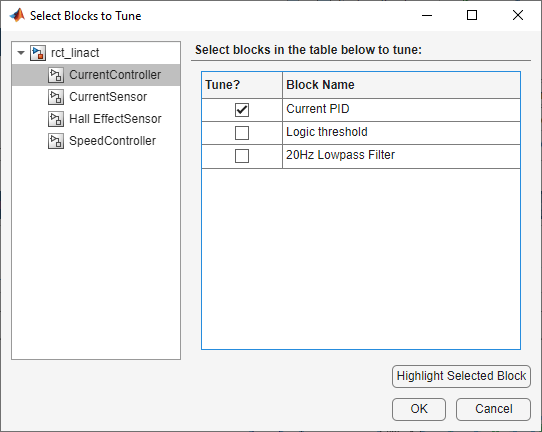Figure 9: Selecting Tuned Block `Current PID`.Figure 10: Selecting Tuned Block `Speed PID`.

Selected tuned blocks `Current PID` and `Speed PID` show in the editor for tuned blocks.Figure 11: Editor Updated with Selected Tuned Blocks.

They also appear in the `Tuned Blocks` section of Data Browser on the left side of Control System Tuner.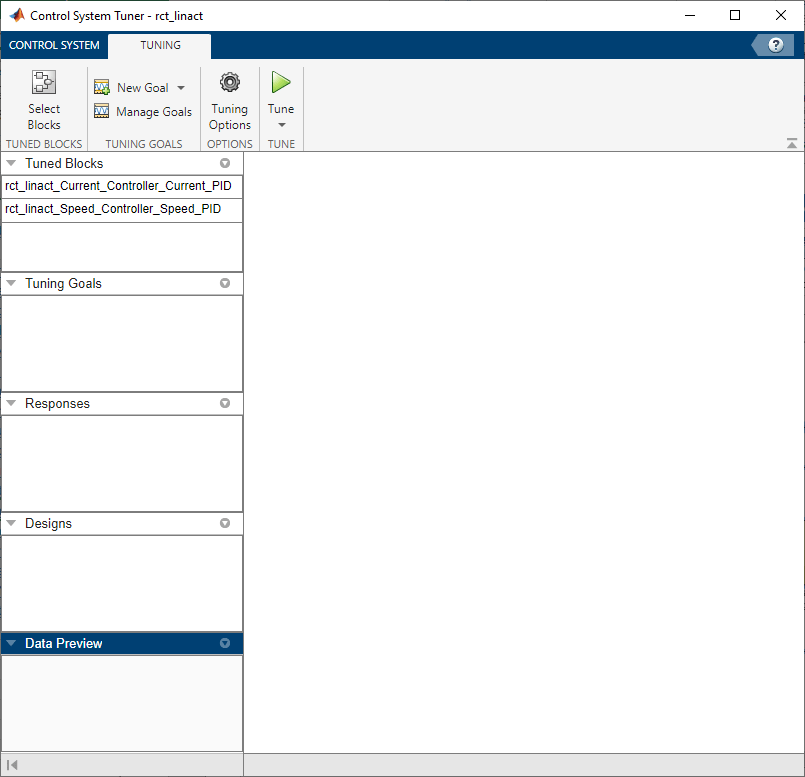Figure 12: Updated Tuned Blocks in Control System Tuner.

Next specify the tracking goal that the DC motor should follow a 2000 rpm speed demand in 0.1 seconds. See different types of goals under `New Goal` and select `Reference Tracking`.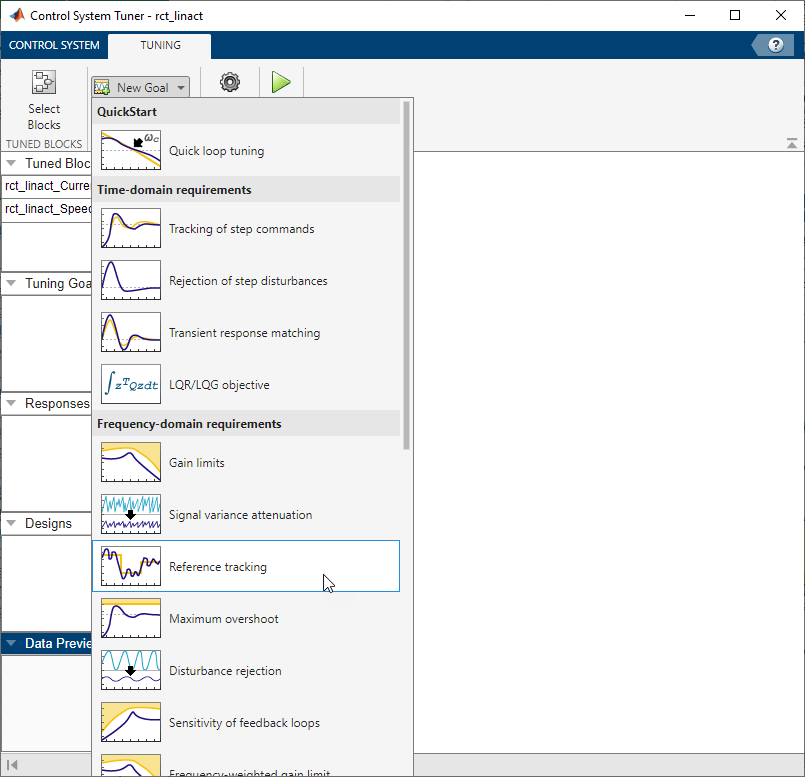Figure 13: Available Goals for Selection in Control System Tuner.

Name the tracking goal as `TR`, specify the tracking goal from the reference input `rct_linact/Speed Demand(rpm)/1` to the reference-tracking output `rct_linact/Hall Effect Sensor/1[rpm]` with the response time 0.1 seconds.The plot for specified tracking goal appears in Control System Tuner and `Tuning Goals` section of Data Browser on the left side is updated.Figure 15: Tracking Tuning Goal in Control System Tuner.

You can now tune the proportional and integral gains with Control System Tuner from clicking `Tune` button. The plot for tracking goal is updated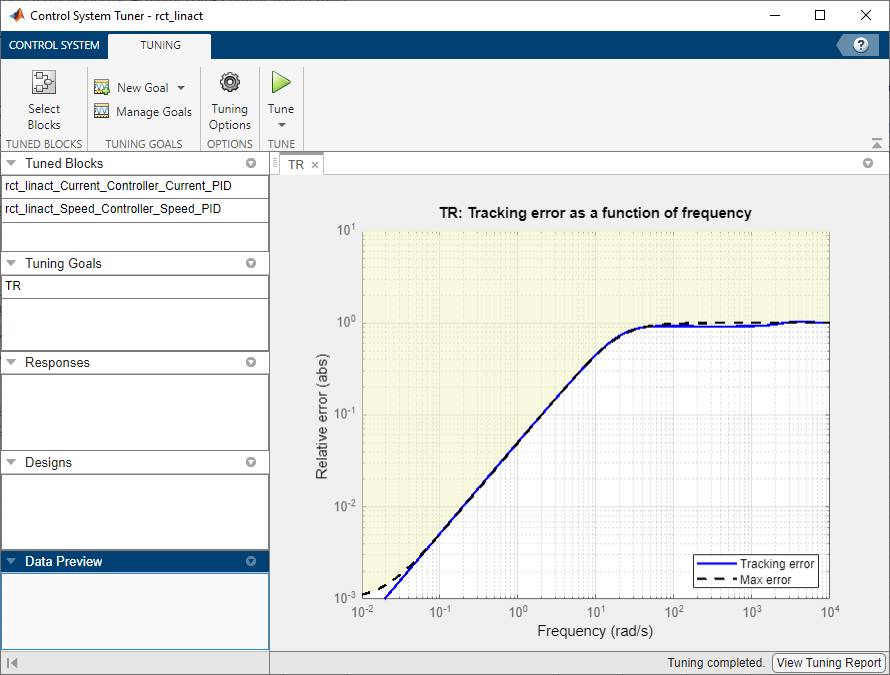Figure 16: Updated Tracking Goal Plot with Tuned Blocks in Control System Tuner.

Tuned blocks are updated with the tuned gain values. To validate this design, plot the closed-loop response from speed demand to speed from `New Plot` of `Control System` Tab.Figure 17: New Plot in Control System Tuner.

Specify the closed-loop response from speed demand to speed by the step plot dialog.You see the step plot of the response in Control System Tuner.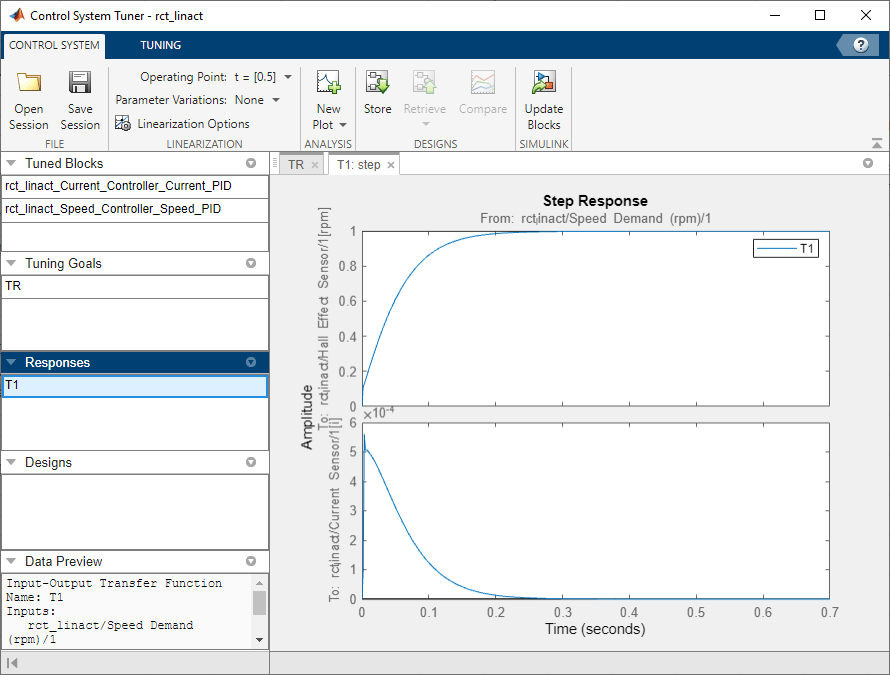Figure 19: Step Plot in Control System Tuner.

The response looks good in the linear domain so first store the current design by clicking `Store` and push the tuned gain values to Simulink by clicking `Update Blocks` and further validate the design in the nonlinear model.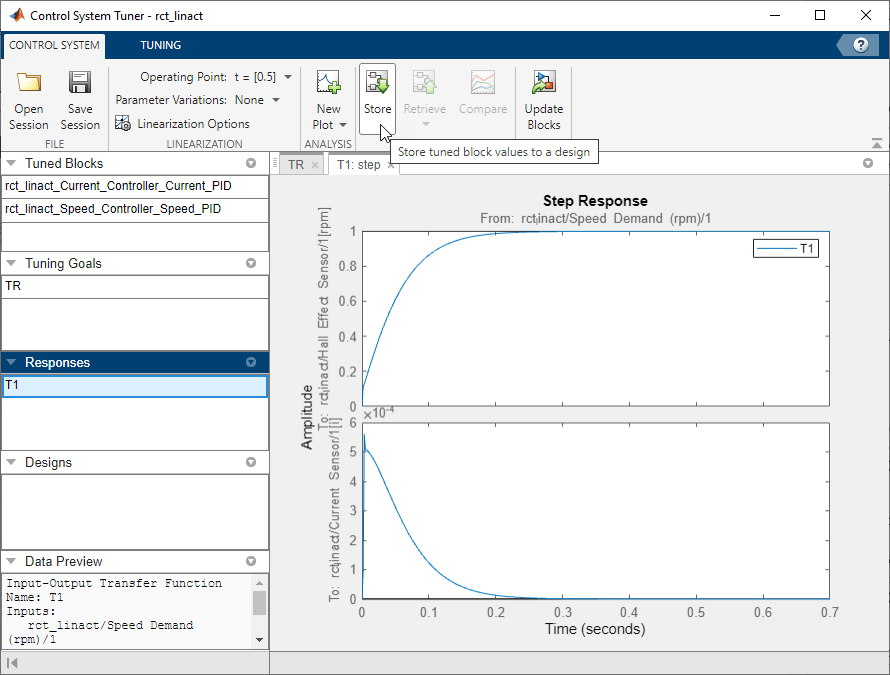Figure 20: Stored Values of Tuned Blocks in Control System Tuner.

The nonlinear simulation results appear in Figure 21. The nonlinear behavior is far worse than the linear approximation, a discrepancy that can be traced to saturations in the inner loop (see Figure 22).Figure 21: Nonlinear Simulation of Tuned Controller.Figure 22: Current Controller Output (limited to plus/minus 5).

### Preventing Saturations

So far we have only specified a desired response time for the outer (speed) loop. This leaves `systune` free to allocate the control effort between the inner and outer loops. Saturations in the inner loop suggest that the proportional gain is too high and that some rebalancing is needed. One possible remedy is to explicitly limit the gain from the speed command to the outputs of the P and PI controllers. For a speed reference of 2000 rpm and saturation limits of plus/minus 5, the average gain should not exceed 5/2000 = 0.0025. To be conservative, we can try to keep the gain from speed reference to controller outputs below 0.001. To do this, add two gain requirements and retune the controller gains with all three requirements in place.

Limit gain from speed demand to control signals to avoid saturation by specifying two new goals from Tuning tab. You need to select control signals from Simulink model since they are not defined previously.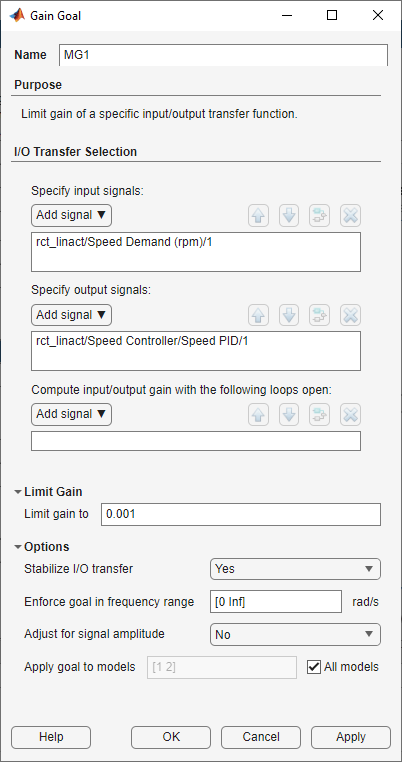Figure 23: Gain Goal Dialog from Speed Demand to Control Signal of Speed PID.Figure 24: Gain Goal Dialog from Speed Demand to Control Signal of Current PID.

New gain goals appear in Tuning Goals section of Control System Tuner.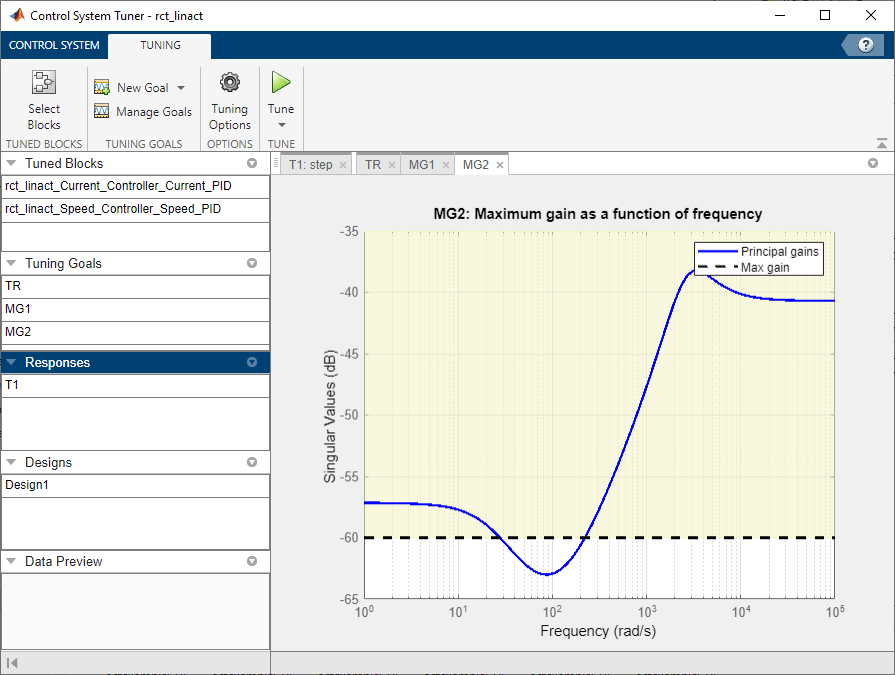Figure 25: Two Gain Goals Added to Control System Tuner.

Retune with these additional requirements. Tuning Report accessed at the bottom right of the tool shows the worst gain 1.39 indicating that the requirements are nearly but not exactly met (all requirements are met when the final gain is less than 1).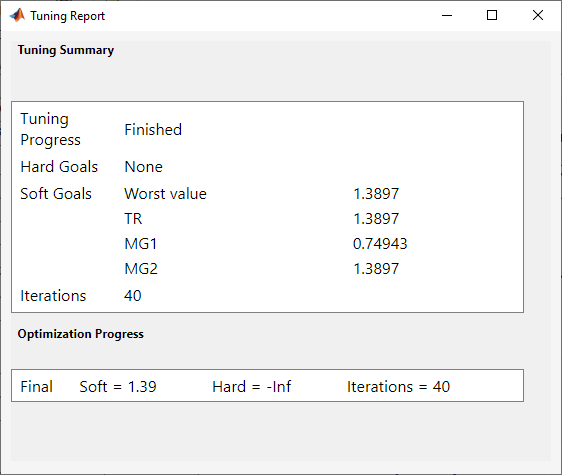Figure 26: Tuning Report After Retuning.

Next compare the two designs in the linear domain by clicking `Compare` in `Control System` tab.Figure 27: Comparing Two Designs.

The second design is less aggressive but still meets the response time requirement.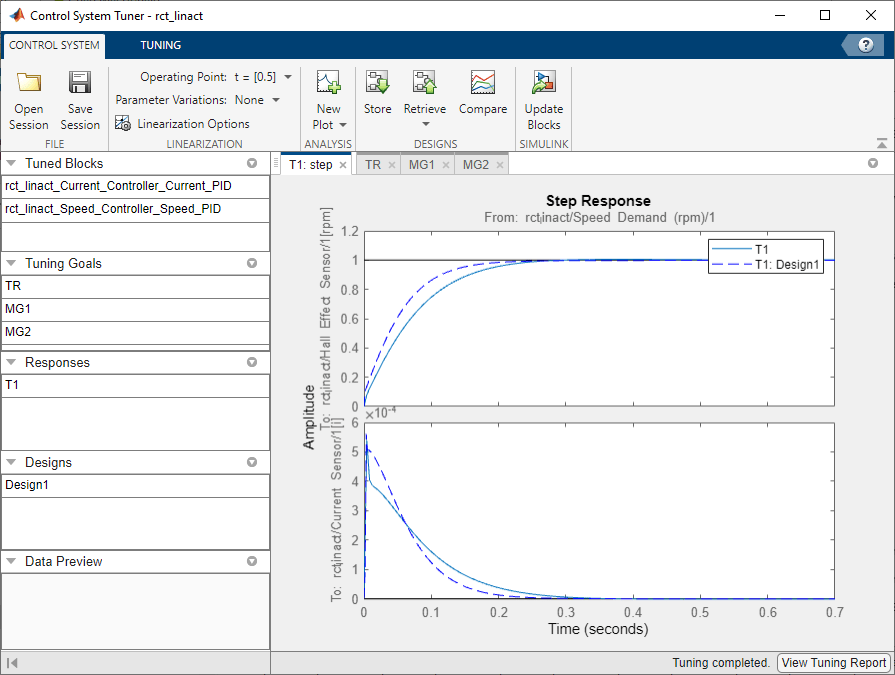Figure 28: Step Responses of Two Designs.

Finally, push the new tuned gain values to the Simulink model by `Update Blocks` and simulate the response to a 2000 rpm speed demand and 500 N load disturbance. The simulation results appear in Figure 29 and the current controller output is shown in Figure 30.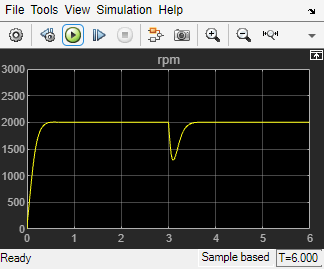Figure 29: Nonlinear Response of Tuning with Gain Constraints.Figure 30: Current Controller Output.

The nonlinear responses are now satisfactory and the current loop is no longer saturating. The additional gain constraints have forced `systune` to re-distribute the control effort between the inner and outer loops so as to avoid saturation.